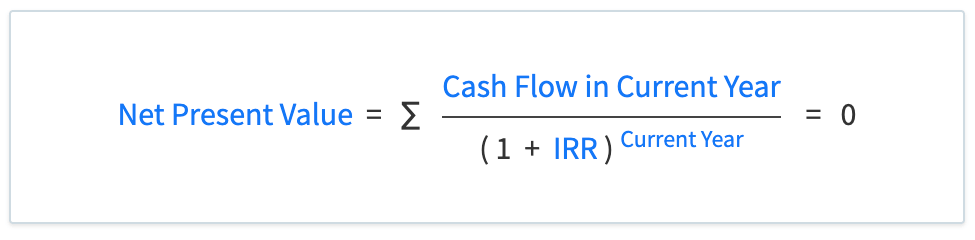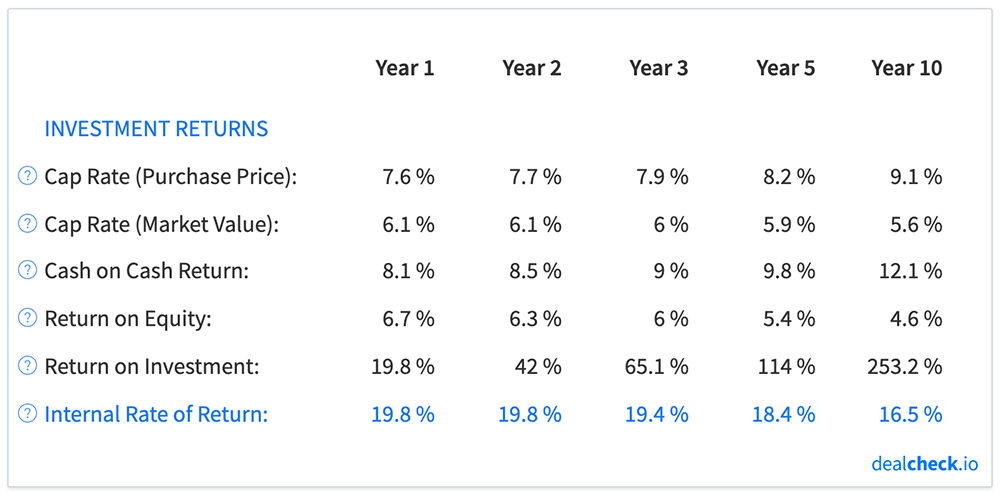The internal rate of return, abbreviated as IRR, is a widely used metric of profitability and a measure of investment return used by real estate investors when evaluating and comparing rental properties and BRRRR’s.

The IRR represents an average annualized return on your invested capital, that takes into account the cumulative cash flow, equity accumulation, loan paydown and sale of a rental property after a specific holding period.

Along with the return on investment (ROI), IRR is often used to compare the profitability of rental properties, as well as to compare the returns of rental properties to other asset classes, such as stocks, bonds, or commodities.

### The Internal Rate of Return (IRR) Formula

Unlike other property analysis metrics, the internal rate of return cannot be calculated algebraically by solving a formula.

Instead, IRR is calculated using trial and error by finding a value that will satisfy the equation below, where the net present value equals \$0:In the formula above, the net present value is calculated as a sum of all discounted cash flows generated by a rental property from the first to the last year of its holding period.

The cash flow in the first year of the holding period is typically negative, as it represents the capital used to purchase and rehab the property (the down payment, purchase and closing costs, rehab costs, etc.).

Subsequent years of property ownership typically generate a stream of positive cash flows, equal to the net cash flow you receive while renting out the property.

The final year of the holding period usually produces a large positive cash flow when you sell the property, pay off any outstanding loans, and collect the final net proceeds after the sale.

### Internal Rate of Return Example

Let’s assume that you purchased a rental property for \$100,000 with a 20% down payment (\$20,000) and paid \$2,000 in closing costs. The home also needed \$15,000 of rehab work to make it rent-ready.

You owned the property for 10 years before selling it for about \$161,000 and paying \$4,800 in closing costs in the process. Throughout the 10 years, you also collected just over \$37,000 of cash flow from this property.

First, let’s calculate how much capital you spent to purchase and rehab this property – your initial cash investment:

Total Invested Cash = Down Payment + Closing Costs + Rehab Costs = \$20,000 + \$2,000 + \$15,000 = \$37,000

Next, let’s calculate how much money you will receive when you sell this property after 10 years – your net sale proceeds:

Sale Proceeds = Sale Price – Loan Balance – Closing Costs = \$161,270 – \$63,027 – \$4,838 = \$93,405

Now, let’s put together a list of all cash flows produced by this property over the 10-year period. This includes the initial investment of \$37,000 (as a negative number, because it was money that you paid), the cash flow collected during each year of property ownership, and the net sale proceeds at the end:And finally, we can take this list of cash flows and apply an iterative approach (which DealCheck automatically does for you) to calculate the internal rate of return for this property over its entire holding period.

IRR = 16.5%

So, what does this number tell you? It means that over a 10 year period, you received an annualized return of 16.5% on the capital you originally invested in this property. Note, that this is not a total return, but instead, an average annual rate of return realized throughout the 10 years.

### Limitations of IRR

While the internal rate of return is a very useful metric for real estate investors, it is not without limitations that you should be aware of.

First, IRR is expressed as a percentage, so it ignores the actual dollar amount of profit (and cash flow) a rental property will generate. Two properties may have the same IRR, but one would net you a \$10,000 total profit over 10 years, while the other – \$100,000. The second property is likely a better investment, but you would not be able to determine that by looking at IRR in isolation.

Next, going along with the above, the IRR does not show you your total cumulative profit from an investment – only an average annualized return. As we discuss in more detail in the next section, it is often useful to know what your total investment return will be and not just an annualized figure.

And finally, because IRR is a time-sensitive metric, the exact cash flows you receive from your rental property, relative to the initial cash investment and the final sales proceeds, may skew the IRR one way or the other, making an investment appear more or less profitable relative to others. Looking at other metrics, and not just the IRR, is the best way to get a more complete picture.

### Internal Rate of Return vs. Return on Investment

The internal rate of return is often calculated and reported along with the return on investment (ROI) or the equity multiple for rental properties and BRRRRs. But what is the difference between these two metrics and which one is more valuable when analyzing potential investment properties?As we’ve discussed earlier, the IRR represents an average annualized return throughout the property holding period, but it does not show your total return on invested capital. So IRR is most useful when you specifically want to compare annualized returns of different properties, or when comparing rental investments to other asset classes.

IRR is also a time-sensitive metric that takes the time value of money into account. If two properties have the same total return (ROI), but one takes 5 years to realize it, while the other takes 10, the first property will have a higher IRR and will likely be a better investment.

On the other hand, ROI shows your total cumulative return on invested capital, regardless of how that return was realized (via cash flow, principal paydown, or property value appreciation) and regardless of how long it took for you to get that return.

ROI is therefore the most useful metric when you want to compare the total cumulative returns and total net profit of different investment properties.

Because IRR and ROI show different rates of return and reveal different insights about a particular investment, it is often helpful to consider both of them together when evaluating and comparing investment properties. While in isolation they show only a partial analysis, together they give a much more complete picture of your projected returns.

### Calculate IRR and Dozens of Other Metrics in Seconds

The DealCheck property analysis app makes it easy to calculate the internal rate of return, along with dozens of other property analysis metrics for rental properties and BRRRR’s in seconds.